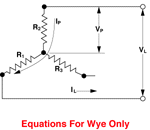INDUSTRIAL PROCESS INSTRUMENTS AND CONTROLS

### Delta and Wye Circuit Equations

Typical 3-Phase Wiring Diagrams and Equations for Resistive Heaters

Definitions

For Both Wye and Delta

VP = Phase Voltage
VL = Line Voltage
IP = Phase Current
IL = Line Current
R = R1 = R2 = R3 = Resistance of each branch
W = Wattage

Wye and Delta Equivalent
WDELTA = 3 WWYE

Open 3-Phase Circuit Formulas:
Open Delta Watts = 2/3 WDELTA
Open Wye Watts = 1/2 WWYE
Open 4-wire Wye Watts = 2/3 WWYE3-Phase Wye (Balanced Load)3-Phase Open Wye (No Neutral)IP = I L VP = VL/1.73 WWYE = VL2 /R = 3 (VP2) /R WWYE = 1.73VLILIPO = I LO VPO = VL/2 WOWYE = 1/2 ( VL /R) WOWYE = 2 (VPO2/R) WOWYE = VLILO3-Phase Delta (Balanced Load) IP = I L/1.73 VP = VL WDELTA = 3(VL2)/R WDELTA = 1.73VLIL3-Phase Open Delta . VP = VL IPO1 = I PO3 = I LO2 ILO3 = 1.73 I PO1 WODELTA = 2(VL2/R )
 Go Shopping Search Products Basket Contents Track Order Company Information Terms & Conditions Your Privacy Manufacturers Contact Us! Technical Library Chemical Compatibility Cleanroom Standards Light Color Codes Conversion Charts Electrical Safety Electrical Symbols FCC Rules Heater Selection Humidity Motors Gears, Drives Ohm's Law Particle Contamination Power Equations Pressure Help Quick Formulas Research Links Temperature Conv t/c  Reference RTD Reference Time Standards 3-Phase Equations LonWorks Carbon Dioxide
 SEARCH  CATALOG Boolean:  AND OR Case Insensitive Sensitive